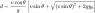# Projectile Range Homework | Help Solve Equation

• rajesh.msen

## Homework Statement

Hi to all guys i am not good in physics and trigonometric I tried to do a projectile system.My launch point is higher than target point.I know the velocity v, target_distance d, gravity g,Launch_height_y y0.I added a image that shows the equation.I tried my own but solved the equation.Please some one help me

d = 30,v = 22, g = 9.8 ,y0 = 2

## The Attempt at a Solution

#### Attachments

•f53a1edde08b3c1c131c0498ea87f8a4.png
1.4 KB · Views: 317
I know the velocity v, target_distance d, gravity g,Launch_height_y y0.I added a image that shows the equation.I tried my own but solved the equation
In this problem, which quantity you want to find out?

i want to find out the angle(theata)